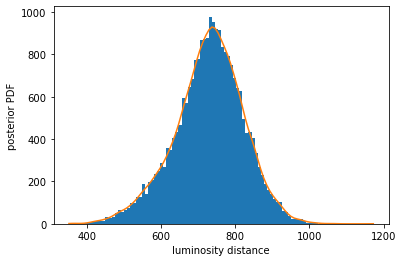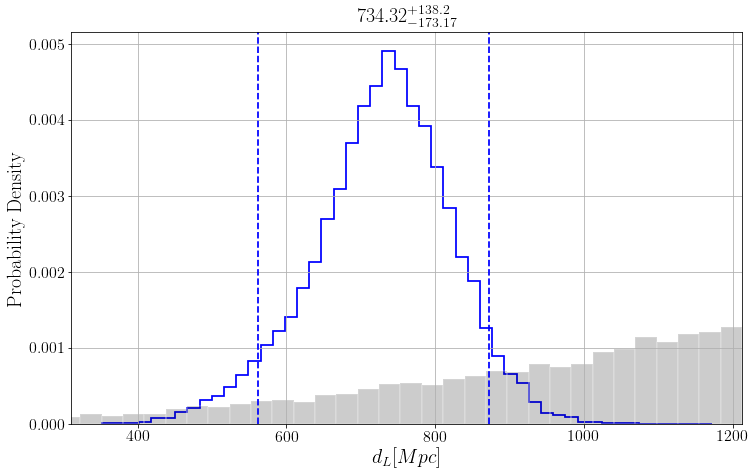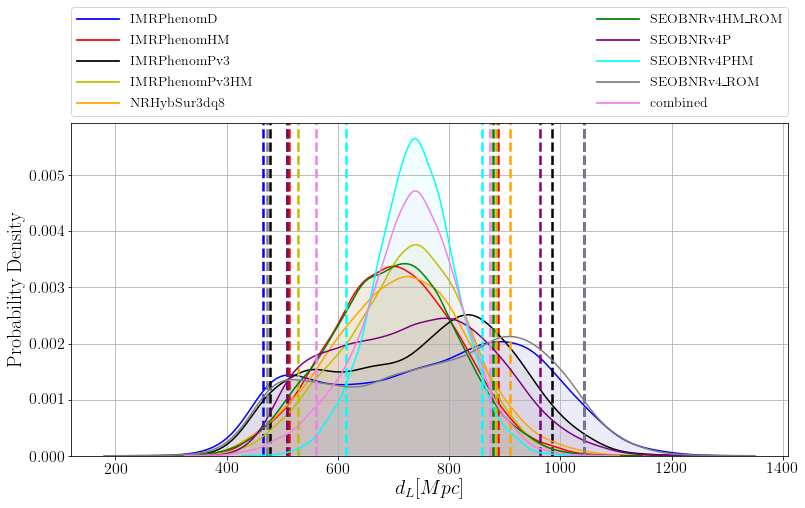# Sample release for GW190412¶

This notebook serves as a basic introduction to loading and viewing data released in associaton with the publication titled GW190412: Observation of a Binary-Black-Hole Coalescence with Asymmetric Masses avaliable through DCC.

The data used in these tutorials can be downloaded from the same DCC page.

The released data file can be read in using the PESummary or h5py libraries. For this notebook we'll start with simple stuff using h5py. Then we'll use PESummary v0.5.1 to read the data files as well as for plotting. For general instructions on how to manipulate the data file and/or read this data file with h5py, see the PESummary docs.

In :
# import useful python packages
%matplotlib inline
import numpy as np
import matplotlib.pyplot as plt
import seaborn as sns
import h5py
import scipy

In :
# There is a known incompatibility between some older versions of numpy/scipy and seaborn.
# Make sure you have an up-to-date version of scipy installed.
# This notebook has been tested with the following versions
for name, package in zip(('numpy', 'scipy', 'seaborn', 'h5py'), (np, scipy, sns, h5py)):
print('{} version: {}'.format(name, package.__version__))

numpy version: 1.17.3
scipy version: 1.3.2
seaborn version: 0.9.0
h5py version: 2.10.0


Some simple stuff with "vanilla" h5py

In :
# read in the data
fn = "posterior_samples.h5"
data = h5py.File(fn,'r')

# print out parametrized waveform family names ("approximants" in LIGO jargon).
print('approximants:',data.keys())

# print out top-level data structures for one approximant. Here fore example we use the combined samples
# between IMRPhenomPv3HM and SEOBNRv4PHM. The data structure is the same for all approximants.
print('Top-level data structures:',data['combined'].keys())

# extract posterior samples for one of the approximants
posterior_samples = data['combined']['posterior_samples']
print('data structures in posterior_samples:',posterior_samples.dtype)
pnames = [item for item in posterior_samples.dtype.names]
print('parameter names:',pnames)

# get samples for one of the parameters
dL = posterior_samples['luminosity_distance']
print('dL shape, mean, std =',dL.shape,dL.mean(),dL.std())

# smooth it
from scipy.stats.kde import gaussian_kde
hs = gaussian_kde(dL)

# histogram, and overlay the smoothed PDF
plt.figure()
h, b, o = plt.hist(dL,bins=100)
hsmoothed = hs(b)*len(dL)*(b-b)
plt.plot(b,hsmoothed)
plt.xlabel('luminosity distance')
plt.ylabel('posterior PDF')
plt.show()

# release memory for the data
#del data

approximants: <KeysViewHDF5 ['IMRPhenomD', 'IMRPhenomHM', 'IMRPhenomPv3', 'IMRPhenomPv3HM', 'NRHybSur3dq8', 'SEOBNRv4HM_ROM', 'SEOBNRv4P', 'SEOBNRv4PHM', 'SEOBNRv4_ROM', 'combined', 'version']>
Top-level data structures: <KeysViewHDF5 ['approximant', 'calibration_envelope', 'config_file', 'injection_data', 'meta_data', 'posterior_samples', 'priors', 'psds', 'version']>
data structures in posterior_samples: [('recalib_L1_amplitude_7', '<f8'), ('recalib_H1_amplitude_9', '<f8'), ('recalib_H1_frequency_3', '<f8'), ('symmetric_mass_ratio', '<f8'), ('spin_1z', '<f8'), ('recalib_L1_phase_3', '<f8'), ('spin_1y', '<f8'), ('recalib_V1_amplitude_2', '<f8'), ('total_mass', '<f8'), ('recalib_V1_amplitude_8', '<f8'), ('recalib_L1_frequency_5', '<f8'), ('recalib_V1_frequency_0', '<f8'), ('recalib_H1_amplitude_1', '<f8'), ('recalib_L1_amplitude_0', '<f8'), ('recalib_L1_frequency_3', '<f8'), ('recalib_L1_frequency_6', '<f8'), ('total_mass_source', '<f8'), ('time_jitter', '<f8'), ('recalib_H1_phase_4', '<f8'), ('chi_2_in_plane', '<f8'), ('recalib_L1_phase_5', '<f8'), ('recalib_H1_amplitude_8', '<f8'), ('final_mass_source', '<f8'), ('V1_matched_filter_abs_snr', '<f8'), ('recalib_H1_frequency_4', '<f8'), ('recalib_L1_frequency_4', '<f8'), ('recalib_V1_phase_2', '<f8'), ('final_spin', '<f8'), ('chi_eff', '<f8'), ('V1_matched_filter_snr', '<f8'), ('recalib_H1_frequency_6', '<f8'), ('recalib_H1_frequency_8', '<f8'), ('recalib_L1_phase_0', '<f8'), ('recalib_L1_frequency_7', '<f8'), ('recalib_V1_amplitude_5', '<f8'), ('recalib_H1_phase_6', '<f8'), ('recalib_H1_amplitude_7', '<f8'), ('V1_matched_filter_snr_angle', '<f8'), ('L1_matched_filter_abs_snr', '<f8'), ('recalib_L1_phase_8', '<f8'), ('chirp_mass_source', '<f8'), ('recalib_V1_amplitude_3', '<f8'), ('recalib_H1_phase_5', '<f8'), ('recalib_V1_phase_3', '<f8'), ('recalib_V1_amplitude_9', '<f8'), ('V1_optimal_snr', '<f8'), ('recalib_L1_phase_4', '<f8'), ('recalib_V1_frequency_9', '<f8'), ('recalib_V1_amplitude_6', '<f8'), ('recalib_V1_amplitude_4', '<f8'), ('final_mass', '<f8'), ('dec', '<f8'), ('recalib_V1_frequency_1', '<f8'), ('recalib_L1_amplitude_9', '<f8'), ('recalib_H1_amplitude_4', '<f8'), ('mass_1', '<f8'), ('recalib_V1_phase_5', '<f8'), ('recalib_H1_frequency_9', '<f8'), ('recalib_V1_phase_0', '<f8'), ('recalib_H1_phase_1', '<f8'), ('log_likelihood', '<f8'), ('recalib_L1_amplitude_6', '<f8'), ('spin_1x', '<f8'), ('recalib_V1_frequency_3', '<f8'), ('mass_1_source', '<f8'), ('phi_2', '<f8'), ('chi_1_in_plane', '<f8'), ('chirp_mass', '<f8'), ('recalib_H1_phase_0', '<f8'), ('recalib_L1_frequency_8', '<f8'), ('recalib_H1_frequency_5', '<f8'), ('recalib_L1_phase_7', '<f8'), ('tilt_2', '<f8'), ('spin_2x', '<f8'), ('ra', '<f8'), ('recalib_H1_frequency_0', '<f8'), ('H1_matched_filter_snr_angle', '<f8'), ('recalib_V1_frequency_6', '<f8'), ('recalib_H1_amplitude_2', '<f8'), ('tilt_1', '<f8'), ('recalib_H1_phase_9', '<f8'), ('recalib_H1_frequency_7', '<f8'), ('network_matched_filter_snr', '<f8'), ('recalib_V1_phase_9', '<f8'), ('cos_theta_jn', '<f8'), ('recalib_V1_phase_6', '<f8'), ('recalib_L1_phase_6', '<f8'), ('recalib_H1_amplitude_6', '<f8'), ('recalib_L1_amplitude_5', '<f8'), ('recalib_H1_amplitude_0', '<f8'), ('L1_matched_filter_snr', '<f8'), ('phi_1', '<f8'), ('H1_optimal_snr', '<f8'), ('recalib_H1_phase_8', '<f8'), ('recalib_V1_phase_1', '<f8'), ('peak_luminosity', '<f8'), ('recalib_V1_frequency_5', '<f8'), ('recalib_H1_phase_7', '<f8'), ('recalib_V1_phase_4', '<f8'), ('recalib_V1_amplitude_0', '<f8'), ('recalib_V1_frequency_8', '<f8'), ('recalib_L1_phase_2', '<f8'), ('recalib_L1_phase_9', '<f8'), ('recalib_L1_amplitude_8', '<f8'), ('iota', '<f8'), ('recalib_V1_amplitude_7', '<f8'), ('recalib_H1_amplitude_5', '<f8'), ('recalib_L1_frequency_9', '<f8'), ('phase', '<f8'), ('geocent_time', '<f8'), ('recalib_L1_amplitude_4', '<f8'), ('cos_tilt_1', '<f8'), ('inverted_mass_ratio', '<f8'), ('mass_ratio', '<f8'), ('theta_jn', '<f8'), ('recalib_L1_frequency_2', '<f8'), ('radiated_energy', '<f8'), ('comoving_distance', '<f8'), ('recalib_L1_frequency_1', '<f8'), ('recalib_V1_amplitude_1', '<f8'), ('recalib_H1_frequency_1', '<f8'), ('recalib_V1_frequency_2', '<f8'), ('phi_jl', '<f8'), ('a_1', '<f8'), ('H1_matched_filter_snr', '<f8'), ('recalib_L1_phase_1', '<f8'), ('recalib_L1_amplitude_1', '<f8'), ('recalib_L1_frequency_0', '<f8'), ('luminosity_distance', '<f8'), ('a_2', '<f8'), ('chi_p', '<f8'), ('redshift', '<f8'), ('recalib_H1_frequency_2', '<f8'), ('cos_tilt_2', '<f8'), ('recalib_V1_frequency_7', '<f8'), ('recalib_V1_phase_8', '<f8'), ('recalib_V1_phase_7', '<f8'), ('recalib_V1_frequency_4', '<f8'), ('recalib_H1_phase_3', '<f8'), ('spin_2y', '<f8'), ('recalib_H1_phase_2', '<f8'), ('H1_matched_filter_abs_snr', '<f8'), ('psi', '<f8'), ('L1_matched_filter_snr_angle', '<f8'), ('cos_iota', '<f8'), ('recalib_L1_amplitude_3', '<f8'), ('recalib_H1_amplitude_3', '<f8'), ('network_optimal_snr', '<f8'), ('spin_2z', '<f8'), ('mass_2_source', '<f8'), ('mass_2', '<f8'), ('phi_12', '<f8'), ('L1_optimal_snr', '<f8'), ('recalib_L1_amplitude_2', '<f8'), ('final_spin_non_evolved', '<f8'), ('peak_luminosity_non_evolved', '<f8'), ('final_mass_non_evolved', '<f8'), ('final_mass_source_non_evolved', '<f8'), ('radiated_energy_non_evolved', '<f8'), ('V1_time', '<f8'), ('H1_time', '<f8'), ('L1_time', '<f8')]
parameter names: ['recalib_L1_amplitude_7', 'recalib_H1_amplitude_9', 'recalib_H1_frequency_3', 'symmetric_mass_ratio', 'spin_1z', 'recalib_L1_phase_3', 'spin_1y', 'recalib_V1_amplitude_2', 'total_mass', 'recalib_V1_amplitude_8', 'recalib_L1_frequency_5', 'recalib_V1_frequency_0', 'recalib_H1_amplitude_1', 'recalib_L1_amplitude_0', 'recalib_L1_frequency_3', 'recalib_L1_frequency_6', 'total_mass_source', 'time_jitter', 'recalib_H1_phase_4', 'chi_2_in_plane', 'recalib_L1_phase_5', 'recalib_H1_amplitude_8', 'final_mass_source', 'V1_matched_filter_abs_snr', 'recalib_H1_frequency_4', 'recalib_L1_frequency_4', 'recalib_V1_phase_2', 'final_spin', 'chi_eff', 'V1_matched_filter_snr', 'recalib_H1_frequency_6', 'recalib_H1_frequency_8', 'recalib_L1_phase_0', 'recalib_L1_frequency_7', 'recalib_V1_amplitude_5', 'recalib_H1_phase_6', 'recalib_H1_amplitude_7', 'V1_matched_filter_snr_angle', 'L1_matched_filter_abs_snr', 'recalib_L1_phase_8', 'chirp_mass_source', 'recalib_V1_amplitude_3', 'recalib_H1_phase_5', 'recalib_V1_phase_3', 'recalib_V1_amplitude_9', 'V1_optimal_snr', 'recalib_L1_phase_4', 'recalib_V1_frequency_9', 'recalib_V1_amplitude_6', 'recalib_V1_amplitude_4', 'final_mass', 'dec', 'recalib_V1_frequency_1', 'recalib_L1_amplitude_9', 'recalib_H1_amplitude_4', 'mass_1', 'recalib_V1_phase_5', 'recalib_H1_frequency_9', 'recalib_V1_phase_0', 'recalib_H1_phase_1', 'log_likelihood', 'recalib_L1_amplitude_6', 'spin_1x', 'recalib_V1_frequency_3', 'mass_1_source', 'phi_2', 'chi_1_in_plane', 'chirp_mass', 'recalib_H1_phase_0', 'recalib_L1_frequency_8', 'recalib_H1_frequency_5', 'recalib_L1_phase_7', 'tilt_2', 'spin_2x', 'ra', 'recalib_H1_frequency_0', 'H1_matched_filter_snr_angle', 'recalib_V1_frequency_6', 'recalib_H1_amplitude_2', 'tilt_1', 'recalib_H1_phase_9', 'recalib_H1_frequency_7', 'network_matched_filter_snr', 'recalib_V1_phase_9', 'cos_theta_jn', 'recalib_V1_phase_6', 'recalib_L1_phase_6', 'recalib_H1_amplitude_6', 'recalib_L1_amplitude_5', 'recalib_H1_amplitude_0', 'L1_matched_filter_snr', 'phi_1', 'H1_optimal_snr', 'recalib_H1_phase_8', 'recalib_V1_phase_1', 'peak_luminosity', 'recalib_V1_frequency_5', 'recalib_H1_phase_7', 'recalib_V1_phase_4', 'recalib_V1_amplitude_0', 'recalib_V1_frequency_8', 'recalib_L1_phase_2', 'recalib_L1_phase_9', 'recalib_L1_amplitude_8', 'iota', 'recalib_V1_amplitude_7', 'recalib_H1_amplitude_5', 'recalib_L1_frequency_9', 'phase', 'geocent_time', 'recalib_L1_amplitude_4', 'cos_tilt_1', 'inverted_mass_ratio', 'mass_ratio', 'theta_jn', 'recalib_L1_frequency_2', 'radiated_energy', 'comoving_distance', 'recalib_L1_frequency_1', 'recalib_V1_amplitude_1', 'recalib_H1_frequency_1', 'recalib_V1_frequency_2', 'phi_jl', 'a_1', 'H1_matched_filter_snr', 'recalib_L1_phase_1', 'recalib_L1_amplitude_1', 'recalib_L1_frequency_0', 'luminosity_distance', 'a_2', 'chi_p', 'redshift', 'recalib_H1_frequency_2', 'cos_tilt_2', 'recalib_V1_frequency_7', 'recalib_V1_phase_8', 'recalib_V1_phase_7', 'recalib_V1_frequency_4', 'recalib_H1_phase_3', 'spin_2y', 'recalib_H1_phase_2', 'H1_matched_filter_abs_snr', 'psi', 'L1_matched_filter_snr_angle', 'cos_iota', 'recalib_L1_amplitude_3', 'recalib_H1_amplitude_3', 'network_optimal_snr', 'spin_2z', 'mass_2_source', 'mass_2', 'phi_12', 'L1_optimal_snr', 'recalib_L1_amplitude_2', 'final_spin_non_evolved', 'peak_luminosity_non_evolved', 'final_mass_non_evolved', 'final_mass_source_non_evolved', 'radiated_energy_non_evolved', 'V1_time', 'H1_time', 'L1_time']
dL shape, mean, std = (23984,) 728.7827424719771 93.20707815487904Now use PESummary v0.5.1 to read the data files as well as for plotting.

In :
# import ligo-specific python packages.
# pesummary is a ligo-specific python package for reading and plotting the results of Bayesian parameter estimation.
# Install with "pip install pesummary" , and make sure you have version >= 0.3.0.
import pesummary
print(pesummary.__version__)

0.5.1: CLEAN: All modifications committed fe78e71


There are 9 different approximants that were used to analyze GW190412 plus the combined samples of IMRPhenomPv3HM and SEOBNRv4PHM. They are all stored in the data file.

In :
fn = "posterior_samples.h5"
labels = data.labels
print(labels)

['IMRPhenomD', 'IMRPhenomHM', 'IMRPhenomPv3', 'IMRPhenomPv3HM', 'NRHybSur3dq8', 'SEOBNRv4HM_ROM', 'SEOBNRv4P', 'SEOBNRv4PHM', 'SEOBNRv4_ROM', 'combined']


To illustrate the data structure we pick the combined posteriors and plot the respective data.

In :
samples_dict = data.samples_dict
posterior_samples = samples_dict["combined"]
prior_samples = data.priors["samples"]["combined"]
parameters = posterior_samples.keys()
print(parameters)

dict_keys(['recalib_L1_amplitude_7', 'recalib_H1_amplitude_9', 'recalib_H1_frequency_3', 'symmetric_mass_ratio', 'spin_1z', 'recalib_L1_phase_3', 'spin_1y', 'recalib_V1_amplitude_2', 'total_mass', 'recalib_V1_amplitude_8', 'recalib_L1_frequency_5', 'recalib_V1_frequency_0', 'recalib_H1_amplitude_1', 'recalib_L1_amplitude_0', 'recalib_L1_frequency_3', 'recalib_L1_frequency_6', 'total_mass_source', 'time_jitter', 'recalib_H1_phase_4', 'chi_2_in_plane', 'recalib_L1_phase_5', 'recalib_H1_amplitude_8', 'final_mass_source', 'V1_matched_filter_abs_snr', 'recalib_H1_frequency_4', 'recalib_L1_frequency_4', 'recalib_V1_phase_2', 'final_spin', 'chi_eff', 'V1_matched_filter_snr', 'recalib_H1_frequency_6', 'recalib_H1_frequency_8', 'recalib_L1_phase_0', 'recalib_L1_frequency_7', 'recalib_V1_amplitude_5', 'recalib_H1_phase_6', 'recalib_H1_amplitude_7', 'V1_matched_filter_snr_angle', 'L1_matched_filter_abs_snr', 'recalib_L1_phase_8', 'chirp_mass_source', 'recalib_V1_amplitude_3', 'recalib_H1_phase_5', 'recalib_V1_phase_3', 'recalib_V1_amplitude_9', 'V1_optimal_snr', 'recalib_L1_phase_4', 'recalib_V1_frequency_9', 'recalib_V1_amplitude_6', 'recalib_V1_amplitude_4', 'final_mass', 'dec', 'recalib_V1_frequency_1', 'recalib_L1_amplitude_9', 'recalib_H1_amplitude_4', 'mass_1', 'recalib_V1_phase_5', 'recalib_H1_frequency_9', 'recalib_V1_phase_0', 'recalib_H1_phase_1', 'log_likelihood', 'recalib_L1_amplitude_6', 'spin_1x', 'recalib_V1_frequency_3', 'mass_1_source', 'phi_2', 'chi_1_in_plane', 'chirp_mass', 'recalib_H1_phase_0', 'recalib_L1_frequency_8', 'recalib_H1_frequency_5', 'recalib_L1_phase_7', 'tilt_2', 'spin_2x', 'ra', 'recalib_H1_frequency_0', 'H1_matched_filter_snr_angle', 'recalib_V1_frequency_6', 'recalib_H1_amplitude_2', 'tilt_1', 'recalib_H1_phase_9', 'recalib_H1_frequency_7', 'network_matched_filter_snr', 'recalib_V1_phase_9', 'cos_theta_jn', 'recalib_V1_phase_6', 'recalib_L1_phase_6', 'recalib_H1_amplitude_6', 'recalib_L1_amplitude_5', 'recalib_H1_amplitude_0', 'L1_matched_filter_snr', 'phi_1', 'H1_optimal_snr', 'recalib_H1_phase_8', 'recalib_V1_phase_1', 'peak_luminosity', 'recalib_V1_frequency_5', 'recalib_H1_phase_7', 'recalib_V1_phase_4', 'recalib_V1_amplitude_0', 'recalib_V1_frequency_8', 'recalib_L1_phase_2', 'recalib_L1_phase_9', 'recalib_L1_amplitude_8', 'iota', 'recalib_V1_amplitude_7', 'recalib_H1_amplitude_5', 'recalib_L1_frequency_9', 'phase', 'geocent_time', 'recalib_L1_amplitude_4', 'cos_tilt_1', 'inverted_mass_ratio', 'mass_ratio', 'theta_jn', 'recalib_L1_frequency_2', 'radiated_energy', 'comoving_distance', 'recalib_L1_frequency_1', 'recalib_V1_amplitude_1', 'recalib_H1_frequency_1', 'recalib_V1_frequency_2', 'phi_jl', 'a_1', 'H1_matched_filter_snr', 'recalib_L1_phase_1', 'recalib_L1_amplitude_1', 'recalib_L1_frequency_0', 'luminosity_distance', 'a_2', 'chi_p', 'redshift', 'recalib_H1_frequency_2', 'cos_tilt_2', 'recalib_V1_frequency_7', 'recalib_V1_phase_8', 'recalib_V1_phase_7', 'recalib_V1_frequency_4', 'recalib_H1_phase_3', 'spin_2y', 'recalib_H1_phase_2', 'H1_matched_filter_abs_snr', 'psi', 'L1_matched_filter_snr_angle', 'cos_iota', 'recalib_L1_amplitude_3', 'recalib_H1_amplitude_3', 'network_optimal_snr', 'spin_2z', 'mass_2_source', 'mass_2', 'phi_12', 'L1_optimal_snr', 'recalib_L1_amplitude_2', 'final_spin_non_evolved', 'peak_luminosity_non_evolved', 'final_mass_non_evolved', 'final_mass_source_non_evolved', 'radiated_energy_non_evolved', 'V1_time', 'H1_time', 'L1_time'])


As an example, we'll show the different posterior distributions derived for a single waveform and the posterior distribution derived using the different approximants for the luminosity_distance parameter.

In :
from pesummary.core.plots.plot import _1d_histogram_plot
from pesummary.gw.plots.latex_labels import GWlatex_labels

parameter = "luminosity_distance"
latex_label = GWlatex_labels[parameter]

fig = _1d_histogram_plot(
parameter, posterior_samples[parameter], latex_label, prior=prior_samples[parameter]
)
fig.set_size_inches(12, 8)
plt.show()In :
sanitized_labels = [l.replace('_', '\_') for l in labels]

In :
from pesummary.core.plots.plot import _1d_comparison_histogram_plot

samples = []
for label in labels:
samples.append(samples_dict[label][parameter])

colors = ['b', 'r', 'k', 'y', 'orange', 'g','purple','cyan','grey','violet']
fig = _1d_comparison_histogram_plot(parameter, samples, colors, latex_label, sanitized_labels, kde=True)
fig.set_size_inches(12, 8)
plt.show()Make a corner plot:

In :
from pesummary.gw.plots.plot import _make_corner_plot

fig = _make_corner_plot(posterior_samples, GWlatex_labels)
plt.show()
#plt.savefig(fn+'_corner.png')